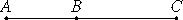# Proposition 37

If two medial straight lines commensurable in square only and containing a rational rectangle are added together, the whole is irrational; let it be called the first bimedial straight line.

Let two medial straight lines AB and BC commensurable in square only and containing a rational rectangle be added together.

I say that the whole AC is irrational.Since AB is incommensurable in length with BC, therefore the sum of the squares on AB and BC is also incommensurable with twice the rectangle AB by BC, and, taken jointly, the sum of the squares on AB and BC together with twice the rectangle AB by BC, that is, the square on AC, is incommensurable with the rectangle AB by BC.

X.Def.I.4

But the rectangle AB by BC is rational, for, by hypothesis, AB and BC are straight lines containing a rational rectangle, therefore the square on AC is irrational. Therefore AC is irrational. And let it be called a first bimedial straight line.

Q.E.D.

## Guide

This proposition is used in X.43, X.55, X.61, and X.67.